# MCAT Physical : Real Gases and Ideal Gases

## Example Questions

### Example Question #1 : Real Gases And Ideal Gases

Under what conditions will a gas most closely follow ideal behavior?

High pressure and low temperature

High pressure and high temperature

Low pressure and high temperature

Low pressure and low temperature

Moderate levels of both temperature and pressure

Low pressure and high temperature

Explanation:

Ideal gases are assumed to have no intermolecular forces and to be composed of particles with no volume. Under high pressure, gas particles are forced closer together and intermolecular forces become a factor. In low temperatures intermolecular forces also increase, since molecules move more slowly, similar to what would occur in a liquid state. Just remember that ideal gas behavior is most closely approximated in conditions that favor gas formation in the first place—heat and low pressure.

### Example Question #1 : Real Gases

Which of the following assumptions is not made by the ideal gas law?

The molecules obey Newton's laws of motion at all times

The molecules move randomly

The size of the molecules is much smaller than the container

The van der Waals forces are negligible

The intermolecular interactions follow the Coulomb model of electric repulsion

The intermolecular interactions follow the Coulomb model of electric repulsion

Explanation:

Under the ideal gas law, we assume that the interactions between the molecules are very brief and that the forces involved are negligible. The assumption that the molecules obey Coulomb's law when interacting with each other is not necessary; rather, an ideal gas must disregard Coulomb's law.

The ideal gas law assumes only Newtonian mechanics, disregarding any intermolecular or electromagnetic forces.

### Example Question #3 : Real Gases And Ideal Gases

Which of the following factors does not explain why measurements of real gases deviate from ideal values?

The volume of the gas particles themselves

Intermolecular forces

The volume between the gas particles

All of these factors will cause deviation from ideal values

The volume between the gas particles

Explanation:

Measurements of real gases deviate from ideal gas predictions because intermolecular forces and the volume of the particles themselves are not taken into consideration for ideal gases. The volume of the space between particles is considered for ideal gases and does not contribute to deviation from ideal gas behavior.

Attraction between molecules causes real pressure to be slightly less than ideal pressure, while the volume of gas particles causes real volume to be slightly greater than ideal volume.

### Example Question #4 : Real Gases And Ideal Gases

The definition of ideal pressure for a real gas is given as follows: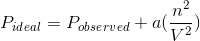The constantsignifies the attraction coefficient. This value is positive if the molecules attract each other and is negative if the molecules repel each other.

Which of the following would have a negative attraction coefficient,, if found in the gaseous state?

Sodium chloride

Magnesium with an electron configuration of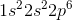Dichloromethane

Oxygen with an electron configuration of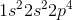Magnesium with an electron configuration ofExplanation:

The question wants you to pick a molecule that will have a negative attraction coefficient,. The question states that the molecules that repel each other will have a negative. Recall that similar charges repel each other; therefore, you are looking for an ionized molecule (molecule with a positive or negative charge). In a closed container, these molecules will be force into contact with each other and generate repulsion forces.

Dichloromethane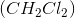is a neutral molecule. This means that the dichloromethane molecules won’t repel each other. Similarly, sodium chloride is a neutral molecule and will not experience repulsion. Oxygen, with an electron configuration of, is also a neutral molecule. If you look at the periodic table, the neutral state of oxygen occurs when there are six valence electrons. The outermost shell of oxygen in this electron configuration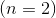has a total of six electrons; therefore, the oxygen has six valence electrons and is neutral.

On the other hand, magnesium, with an electron configuration of, is not neutral. Recall that a neutral magnesium atom has two valence electrons and an electron configuration of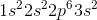. The magnesium atom in this question, however, has lost two electrons (from the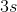orbital) and became positively charged with a charge of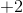; therefore, the magnesium atoms are ionized, will repel each other, and will have a negative.

### Example Question #2 : Real Gases

Consider a real gas with a constant amount and a constant pressure. It has a temperature of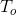and a volume of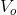. If you double the temperature, what will happen to the volume?

The volume will become greater than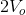The volume will become less thanThe volume will becomeThe volume will become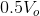The volume will become less thanExplanation:

This question can be solved using either Charles's law or the ideal gas law (converted into the combined gas law).

Charles's Law: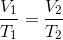Ideal Gas Law: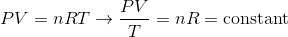The question states that the pressure and moles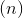are held constant; therefore, the volume and temperature are directly proportional. If the question were asking about an ideal gas, the volume would double when you double the temperature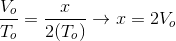The volume would double for an ideal gas; however, the question is asking about a real gas. To find the correct relationship between volume and temperature we need to look at the equation for real gas volume. Remember that the volume we are concerned with is the volume of the free space in the container, given by the container volume minus the volume of the gas particles. The equation for real gas volume accounts for the volume of the container and the volume of the gas particles. For a real gas, the volume is given as follows: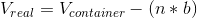In this equation,is the number of moles of gas particles andis the bigness coefficient. This equation implies that the volume of free space for a real gas is always less than the volume for an ideal gas; therefore, doubling the temperature will produce a volume that is less than the predicted volume for an ideal gas. Our answer, then, must be less than double the initial volume.

Note that for an ideal gas the bigness coefficient,, would be zero and the volume of free space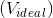would be equal to the volume of the container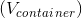. This occurs because the volume of the gas particles is negligible for an ideal gas.

### Example Question #1 : Real Gases And Ideal Gases

Which of the following gases would behave the least ideally?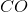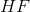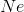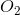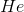Explanation:

For a gas to behave ideally, is should have a low mass and/or weak intermolecular forces. Contrastingly, non-ideal gasses should have very large masses and/or have strong intermolecular forces. Therefore, the correct answer iswhich has very strong intermolecular forces, hydrogen bonds. Nonpolar gasses such as oxygen, and other diatomic gasses have very weak intermolecular forces and thus behave ideally.

### Example Question #3 : Real Gases

Which of the following is relevant for real gases, but irrelevant for ideal gases?

I. Volume of gas particles

II. Intermolecular forces between gas particles

III. Volume of container

I only

I and III

I and II

III only